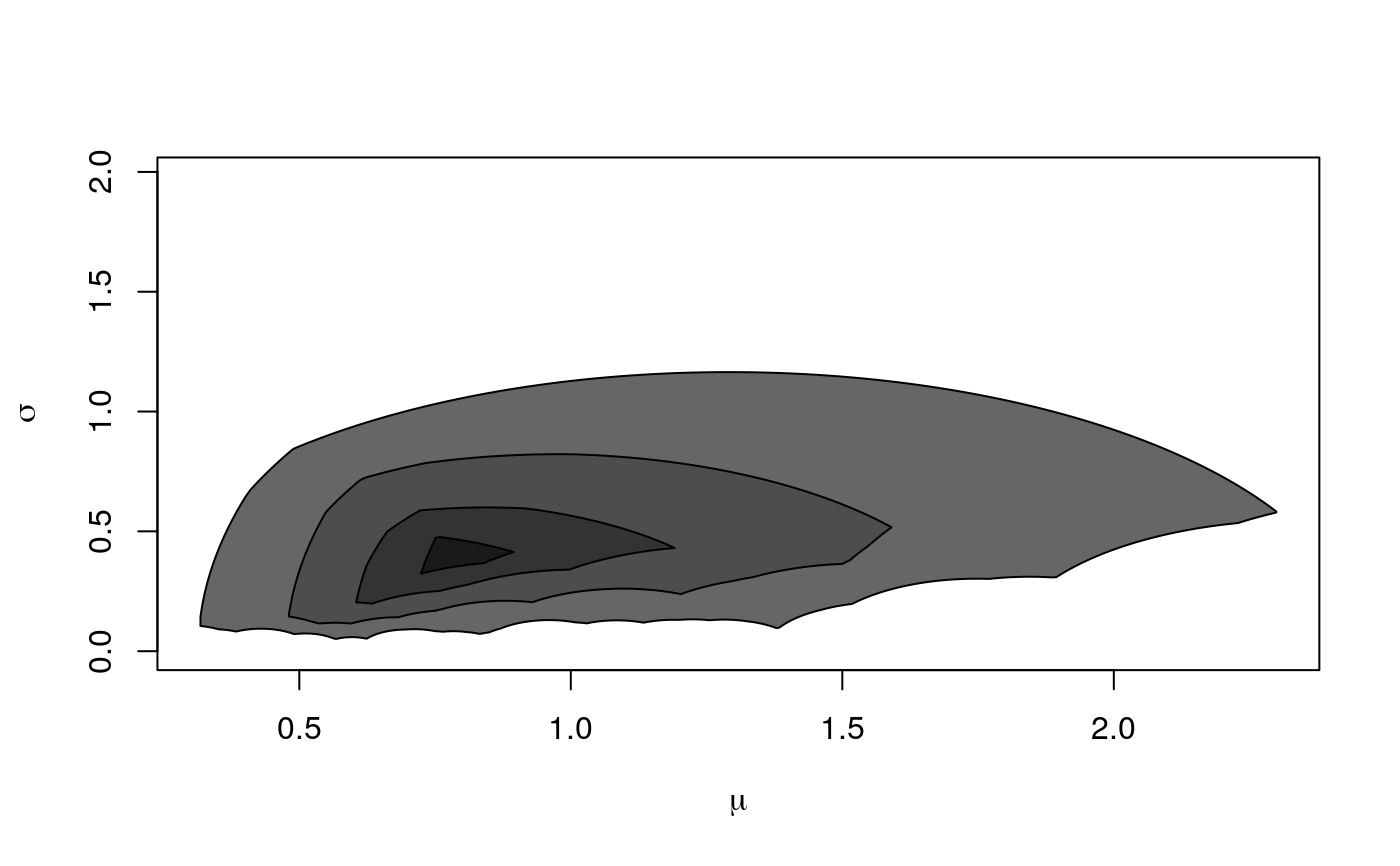Create location-scale depth plot. See lsdSampleDepthContours for more information.

# S4 method for LSDepthContour,ANY
plot(x, cont = NULL, ratio = 1,
mu_min = NULL, mu_max = NULL, col = NULL, border = NULL, ...)

## Arguments

x object of class LSDepthContour plotted contours. Default NULL means that all contours stored in x will be plotted. ratio mu_min mu_max vectors with area colors passed to polygon function vector with colors for borders other parameters passed to polygon

## Examples


smp <- rf(100, 5, 10)
x <- lsdSampleDepthContours(smp)
plot(x, col = paste0("grey", col = rev(seq(10, 40, 10))))